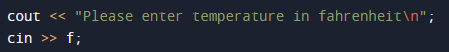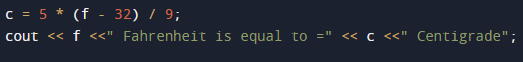# C++ Program to Convert Fahrenheit to Celsius

In this tutorial you will learn about the C++ Program to Convert Fahrenheit to Celsius and its application with practical example.

In this tutorial, we will learn to create a c++ program that Convert Fahrenheit to Celsius using c++ programming

## Prerequisites

Before starting with this tutorial we assume that you are best aware of the following C++ programming topics:

• Operators.
• looping statements.
• Basic input/output.
• Basic c++ programming
• For Loop.
• String.

## What Is Temperature ?

Temperature is a quantity that measured the freezing and boiling point of water. Which is measured in different scales like Celsius and Fahrenheit.
In Celsius scale, the boiling point of water is 100°C, and the freezing point is at 0°C, while in the Fahrenheit scale the boiling point of water is 212°F and freezing point is at 32°F.

## C++ Program to Convert Fahrenheit to Celsius.

In this program we will convert temperature from Fahrenheit to Celsius. We would first declared and initialized the required variables. Next, we would prompt user to input the value in Fahrenheit. Later we will Convert the values into Celsius.

To convert values from Fahrenheit to Celsius we will use following Formula:

C = (F – 32)*(5/9)where, F is temperature in Fahrenheit and C is temperature in Celsius.

## Output## Logic to find Fahrenheit to Centigrade

First of all Take the Fahrenheit  temperature in f
Then calculate c = 5 * (f – 32) / 9.
return C.

In the above program, we have first declared and initialized a set variables required in the program.

• f = it will hold Fahrenheit value.
• c= it will hold Celsius value.

And  in the next statement user will be prompted to enter value in Fahrenheit and  which will be assigned to variable ‘f’.After that with the help of  formula we will convert the value in to Celsius.As you can see in above program, we first take value in Fahrenheit  as input from user. Then convert the value of Fahrenheit in to Celsius using above conversion equation and print the Temperature in Celsius.

In this tutorial we have learn about the C++ Program to Convert Fahrenheit to Celsius and its application with practical example. I hope you will like this tutorial.